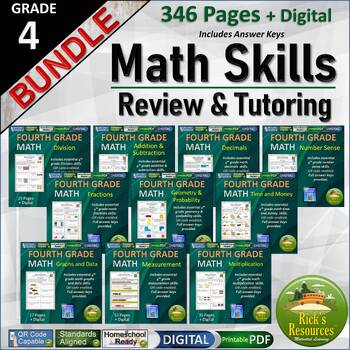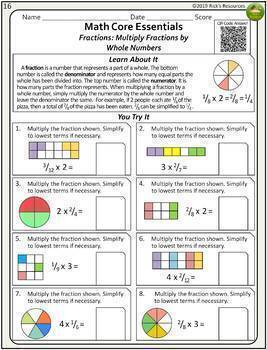4th Grade Math Skills Review and Tutoring Bundle - Print and Digital Versions4th, Homeschool
Subjects
Standards
Resource Type
Formats Included
• Zip
•Google Apps™
Pages
346 Pages + Digital Versions
\$34.25
Bundle
List Price:
\$44.25
You Save:
\$10.00
\$34.25
Bundle
List Price:
\$44.25
You Save:
\$10.00This bundle contains one or more resources with Google apps (e.g. docs, slides, etc.).

Products in this Bundle (10)

showing 1-5 of 10 products

Also included in

1. Printable PDF files are included for all resources. Five resources also include a digital Google Slides™ version for distance learning. Bundled are 29 quality math resources for 4th grade which address important problem-solving, vocabulary, fractions, basic operation skills. Some use QR coding for
\$99.95
\$131.58
Save \$31.63

Description

Printable PDF and digital Google Slides™ versions included for blended learning. These math worksheets effectively address Fourth Grade core math concepts. Useful for practice and review and tutoring applications. Each PDF worksheet is QR coded for instant feedback. Full answer keys provided.

--- RESOURCE DETAILS ---

You are covered with this resource. It includes a PDF printable version as well as a Google Slides™ digital version for each resource. It's great for either in-person class or distance learning at home. The math worksheets address core standards in an effective and motivating format. Each worksheet indicates the skill being practiced and provides short instruction followed by practice problems - making them useful for parent volunteers or tutors. Students can receive instant feedback to their choices by reading the QR code on the PDF worksheet or by accessing the answer keys. Prints well in color or grayscale.

The core concepts addressed in this 4th grade core math kit are as follows:

Number Sense

* Standard and Word Forms

* Standard and Expanded Forms

* Place Value Digit Replacement

* Place Value Digit Value

* Place Value More or Less

* Roman Numerals

* Base 10 Models

* Equality and Inequality

* Comparing Numbers

* Rounding Numbers

* Prime and Composite Numbers

* Even and Odd Numbers

* Estimation

* Patterns

* Commutative Property

* Associative Property

* Addition Fill in the Missing Digits

* Subtract Numbers to Thousands

* Subtract Numbers to Millions

* Subtraction Problem-Solving

* Subtraction Fill in the Missing Digits

* Subtraction Estimating Differences

Multiplication

* Commutative Property of Multiplication

* Associative Property of Multiplication

* Distributive Property of Multiplication

* Multiplication Models

* Multiply 2-digit by 1-digit

* Multiply 3-digit by 1-digit

* Multiply 4-digit by 1-digit

* Multiply 2-digit by 2-digit

* Multiplication Problem-Solving

* Multiplication and Zeros

* Multiplication and Square Numbers

* Multiplication and Estimating Products

* Multiplication and Input/Output Tables

* Lattice Multiplication

Division

* Division Models

* Division Estimating Quotients

* Division Facts W/Without Remainder

* Division 2-digits by 1-digit Level 1

* Division 2-digits by 1-digit Level 2

* Division 3-digits by 1-digit Level 1

* Division 3-digits by 1-digit Level 2

* Division 3-digits by 1-digit Level 3

* Division 3-digits by 1-digit Level 4

* Division 2-digits by 2-digits Level 1

* Division Problem-Solving

* Division Equalities and Inequalities

Graphs and Data

* Coordinate Plane Graphs

* Coordinate Plane Map Graph

* Vertical Bar Graph

* Horizontal Bar Graph

* Pictograph

* Line Graph

* Circle Graph

* Frequency Charts

* Tables

* Stem and Leaf Plots

Time and Money

* Reading Digital & Analog Clocks

* Elapsed Time

* Time Zones

* Time Word Problems

* Coins and Bills

* Multiplying & Dividing Money

* Making Change

* Money Word Problems

Customary and Metric Measurement

* Measuring Length

* Estimating Length

* Converting Length

* Measuring Weight

* Estimating Weight

* Converting Weight

* Measuring Liquid Volume

* Estimating Liquid Volume

* Converting Liquid Volume

* Area

* Perimeter

Fractions

* Identifying Fractions

* Equivalent Fractions

* Patterns of Equivalent Fractions

* Fraction Equality and Inequality

* Ordering Fractions

* Adding Fractions – Like Denominators

* Adding Fractions – Unlike Denominators

* Subtracting Fractions – Like Denominators

* Subtracting Fractions – Unlike Denominators

* Fraction Word Problems*Mixed Numbers and Improper Fractions

* Fractions – Lowest Terms

* Multiplying Fractions

* Multiplying Fractions Word Problems

Decimals

* Decimal Models*Decimal Models with Whole Numbers

* Decimal Place Value

* Decimal Number Line Tenths

* Decimal Number Line Hundredths

* Graphing Points on Number Line

* Equivalent Decimals

* Decimal Word Forms Tenths

* Decimal Word Forms Hundredths

* Converting Fractions to Decimals*Converting Mixed Numbers to Decimals

* Rounding Decimals

* Decimal Equality and Inequality

* Decimals Ordering

* Decimals Subtracting

* Decimals Multiplying

* Decimals Dividing

* Decimal Word Problems

Geometry and Probability and Variables

* Geometry Lines

* Identifying Angles

* Measuring Angles

* Shapes

* Classifying Shapes

* Classifying Triangles

* Perimeter

* Area

* 3D Shapes

* Vertices, Edges, Faces

* Solid Volume

* Line or Reflection Symmetry

* Probability Making Predictions

* Mean, Mode, Median, Range

* Variables – Evaluate Expressions

* Solve Variable Equations

* Expressions with Variables in Stories

--- APPLICATIONS ---

Classroom teachers can use the worksheets to supplement the math program for reviewing and practicing core math skills. Great for review before math tests. Since the worksheets include basic instruction, they can be used for homework or centers and are great for parent volunteers or tutoring. The worksheets can be effective for summer review and for parent monitored home practice.

Rick Whitehead has been a teacher and author for over 30 years in public and private practice. His background includes teaching in public schools, curriculum development, school program design, private mentoring and author of juvenile fiction. He is devoted to helping children learn and grow by providing high-quality resources that are highly motivating and effective even for the most reluctant learners.

Total Pages
346 Pages + Digital Versions
Included
Teaching Duration
N/A
Report this Resource to TpT
Reported resources will be reviewed by our team. Report this resource to let us know if this resource violates TpT’s content guidelines.

Standards

to see state-specific standards (only available in the US).
Compare two decimals to hundredths by reasoning about their size. Recognize that comparisons are valid only when the two decimals refer to the same whole. Record the results of comparisons with the symbols >, =, or <, and justify the conclusions, e.g., by using a visual model.
Use decimal notation for fractions with denominators 10 or 100. For example, rewrite 0.62 as 62/100; describe a length as 0.62 meters; locate 0.62 on a number line diagram.
Express a fraction with denominator 10 as an equivalent fraction with denominator 100, and use this technique to add two fractions with respective denominators 10 and 100. For example, express 3/10 as 30/100, and add 3/10 + 4/100 = 34/100.
Recognize a line of symmetry for a two-dimensional figure as a line across the figure such that the figure can be folded along the line into matching parts. Identify line-symmetric figures and draw lines of symmetry.
Classify two-dimensional figures based on the presence or absence of parallel or perpendicular lines, or the presence or absence of angles of a specified size. Recognize right triangles as a category, and identify right triangles.